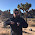## Sunday, March 1, 2015

### Information equilibrium is an equivalence relation

Something for the math nerds. I've said it a couple times, but haven't actually shown the proof. However, it is true that information equilibrium is an equivalence relation. If we define the statement $A$ to be in information equilibrium with $B$ (which we'll denote $A \cong B$) by the relationship (i.e. ideal information transfer from $A$ to $B$):

$$\text{(1) }\;\; \frac{dA}{dB} = k \frac{A}{B}$$

for some value of $k$, then, first we can show that $A \cong A$ because

$$\frac{dA}{dA} = k \frac{A}{A}$$

$$1 = k \cdot 1$$

and we can take $k = 1$. Second we can show that $A \cong B$ implies $B \cong A$ by re-deriving the relationship (1), except moving the variables to the opposite side:

$$\frac{dB}{dA} = \frac{1}{k}\;\; \frac{B}{A} = k' \; \frac{B}{A}$$

for some $k'$ (i.e. $k' = 1/k$). Lastly we can show that $A \cong B$ and $B \cong C$ implies $A \cong C$ via the chain rule:

$$\text{(2a) }\;\; \frac{dA}{dB} = a \frac{A}{B}$$

$$\text{(2b) }\;\; \frac{dB}{dC} = b \frac{B}{C}$$

such that

$$\frac{dA}{dC} = \frac{dA}{dB}\; \frac{dB}{dC} = a b \; \frac{A}{B} \frac{B}{C}$$

$$\frac{dA}{dC} = k \; \frac{A}{C}$$

with information transfer index $k = a b$. That gives us the three properties of an equivalence relation: reflexivity, symmetry and transitivity.

1.Jason, how does it work in the (very) long run?

http://www.bankofengland.co.uk/research/Pages/onebank/datasets.aspx#5

1.It works fairly well on that dataset:

http://informationtransfereconomics.blogspot.com/2013/11/the-long-run-in-uk.html

In looking at that dataset, there were some interesting insights on how the labor market works:

http://informationtransfereconomics.blogspot.com/2013/11/the-labour-supply-part-1.html

http://informationtransfereconomics.blogspot.com/2013/11/the-labour-supply-part-2.html

However, I hadn't quite worked out how the monetary base reserves worked in the model at the time, so I should probably re-do the results.

It also works reasonably well on a long run US dataset:

http://informationtransfereconomics.blogspot.com/2014/09/the-us-economy-1798-to-present.html

The separation into monetary regimes still seems somewhat ad hoc to me, but remains important.

2.Comments are welcome. Please see the Moderation and comment policy.

Also, try to avoid the use of dollar signs as they interfere with my setup of mathjax. I left it set up that way because I think this is funny for an economics blog. You can use € or £ instead.